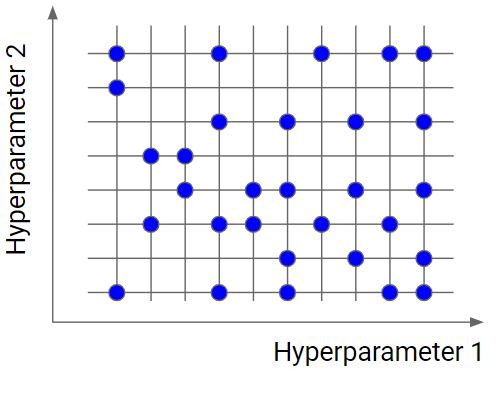# Harnessing the Power of Grid Search for Optimized Machine Learning Models### Introduction

Machine learning is a fast-evolving field, and choosing the optimal parameters for a machine learning algorithm is often a challenging task. This is where hyperparameter tuning methods like Grid Search come into play, proving to be valuable tools for enhancing model performance. This article delves deep into the concept of Grid Search, its role in machine learning, its advantages, and how it operates, providing a comprehensive understanding of this potent optimization technique.

### The Concept of Grid Search

Grid Search is a hyperparameter tuning technique used to identify the ideal parameters that can improve the learning process and accuracy of a machine learning model. Hyperparameters are the configuration settings used to tune the model performance. They are not learned from the data but are set beforehand and influence the learning process.

In essence, Grid Search is an exhaustive search method that works by defining a grid of hyperparameters and evaluating the model’s performance for each point on the grid. You can think of this process as a brute-force method to estimate hyperparameters.

### The Importance of Hyperparameter Tuning in Machine Learning

Before delving into the specifics of Grid Search, it’s crucial to understand the importance of hyperparameters in machine learning. These are the parameters that control the learning process of the model and are set before the learning process begins.

While model parameters are learned during the training process, hyperparameters are not. They need to be preset, and their correct configuration can significantly affect the learning process and model’s performance.

Hyperparameters might include the number of leaves or depth in a decision tree, the number of hidden layers in a neural network, the learning rate in gradient descent, among others. Tuning these parameters accurately can dramatically improve your model’s accuracy and performance.

### Grid Search: The Process

Grid Search operates by constructing a grid of hyperparameter values and evaluating the model’s performance for each combination in this hyperparameter space. This is accomplished through cross-validation, typically k-fold cross-validation, where the data is split into k subsets, and the holdout method is repeated k times.

For every possible combination of hyperparameters on the grid, the following steps are executed:

1. Set the model with the specific combination of hyperparameters.
2. Perform k-fold cross-validation.
3. Calculate the average result.

The combination of hyperparameters that yields the best average result is selected as the optimal solution.

### The Power and Limitations of Grid Search

Grid Search is a powerful tool because it is straightforward, easy to use, and because it conducts an exhaustive search, it can find the optimal hyperparameters that may be missed by other search strategies.

However, Grid Search does have its limitations. The main one being that it can be computationally expensive, especially for large datasets with many parameters. It also assumes that the best parameters are on the grid, and if the grid is not fine enough or does not include the correct range, the optimal parameters might be missed.

Moreover, Grid Search treats each hyperparameter independently, which might not be the best approach if there is interaction between parameters.

### Beyond Grid Search: Random Search and Bayesian Optimization

While Grid Search is a potent technique, other strategies can also be employed for hyperparameter tuning. Random Search and Bayesian Optimization are two such techniques.

Random Search selects random combinations of hyperparameters to train the model, which can be more efficient than Grid Search, especially when dealing with many hyperparameters and large datasets.

Bayesian Optimization, on the other hand, constructs a probability model of the objective function and uses it to select the most promising hyperparameters to evaluate in the actual objective function.

### Conclusion

Grid Search is an invaluable tool in a data scientist’s toolkit, providing an exhaustive mechanism for tuning hyperparameters and optimizing machine learning model performance. Although it comes with its computational costs, the benefit of potentially improving model performance makes it a technique worth considering in the quest for building high-performing machine learning models.

Understanding the nuances of Grid Search, its advantages, limitations, and its alternatives like Random Search and Bayesian Optimization can empower you to make informed decisions and develop efficient machine learning models. The power of machine learning lies not just in complex algorithms but also in the clever strategies used to fine-tune these models, such as Grid Search.

# Personal Career & Learning Guide for Data Analyst, Data Engineer and Data Scientist

## Applied Machine Learning & Data Science Projects and Coding Recipes for Beginners

A list of FREE programming examples together with eTutorials & eBooks @ SETScholars

# Projects and Coding Recipes, eTutorials and eBooks: The best All-in-One resources for Data Analyst, Data Scientist, Machine Learning Engineer and Software Developer

Topics included:Classification, Clustering, Regression, Forecasting, Algorithms, Data Structures, Data Analytics & Data Science, Deep Learning, Machine Learning, Programming Languages and Software Tools & Packages.
(Discount is valid for limited time only)## Find more … …

Machine Learning for Beginners – A Guide to use Grid Search Hyperparameters for Deep Learning Models With Keras in Python

Learn Keras by Example – Tuning Neural Network Hyperparameters

Machine Learning for Beginners – A Guide to Deep Learning (LSTM) Hyperparameters tuning with Keras for Time Series Forecasting in Python